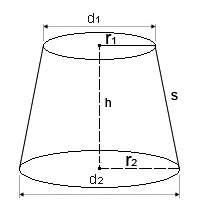# Frustum Calculator

A Frustum can be defined as a geometric figure that consists of three dimensions, and its top has been removed by a plane parallel to the base.

The diameters of the top side and the base are parallel to each other and are located in the centre of the axis.

It is important to recall some basic information that is relevant to the Frustum Calculator. The dimensions that can be found using the calculator include: the vertical height of the figure Frustum, the slope height of the sides, top and base diameters as well as to and base radiuses.

Square units are used for the following dimensions: the top surface area, lateral surface area, base surface area and total surface area. The volume is calculated in units cubed.

Also, the number of decimal places to which the calculations are carried out it preset to be 2, but it can be changed by the user.

Frustum Shape CalculatorYou may set the number of decimal places in the online calculator. By default there are only two decimal places.

Results
Top Surface Area (T): 0.00 square units
Lateral Surface Area (L): 0.00 square units
Base Surface Area (B): 0.00 square units
Total Surface Area (A): 0.00 square units
Volume: 0.00 cubic units

### The Frustum Calculator is capable of applying the following algorithms:

Slope Height (s) = √((r2 - r1)2+h2)

Lateral surface area (L) = π x (r1 + r2) x s

Surface area of top (T) = πr12

Surface area of base (B) = πr22

Total area = T + B + L

Volume = (1/3) x π x h x (r12 + r22 + (r1 * r2))